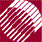ECE532 Biomedical Optics © 1998 Steven L. Jacques, Scott A. Prahl Oregon Graduate Institute

Photon spins into new trajectory due to scattering

The remaining photon weight is now scattered into a new trajectory. If the anisitropy (g) equals 0, then the scattering is isotropic. If g > 0, then the program uses the Henyey-Greenstein scattering function originally introduced by Henyey and Greenstein to mimic the scattering of light from distant stars by galactic dust. The same function approximately mimics the scattering function experimentally observed in biological tissues (see HG function).

The four sections of SPIN are:

• Sample the deflection angle (theta)
The deflection angle (theta) describes how the photon is deflected from its current trajectory. A random number (rnd) selects a choice for the value cos(theta), called costheta. The value sin(theta) called sintheta is also calculated.
• Sample the azimuthal angle (psi)
The deflection is assumed to be directed with equal probability into any azimuthal angle (psi). A random number (rnd) selects a choice for psi which is used to generate values for cos(psi) and sin(psi), called cospsi and sinpsi.
• Calculate the new trajectory.
The new trajectory (uxx, uyy, uzz) is calculated based on costheta, sintheta, cospsi, and sinpsi and on the current trajectory (ux, uy, uz).
• Update current trajectory
The current trajectory is updated, (ux, uy, uz) = (uxx, uyy, uzz).
/**** SPIN
Scatter photon into new trajectory defined by theta and psi.
Theta is specified by cos(theta), which is determined
based on the Henyey-Greenstein scattering function.
Convert theta and psi into cosines ux, uy, uz.
*****/
/* Sample for costheta */
rnd = RandomNum;
if (g == 0.0)
costheta = 2.0*rnd - 1.0;
else {
double temp = (1.0 - g*g)/(1.0 - g + 2*g*rnd);
costheta = (1.0 + g*g - temp*temp)/(2.0*g);
}
sintheta = sqrt(1.0 - costheta*costheta); /* sqrt() is faster than sin(). */

/* Sample psi. */
psi = 2.0*PI*RandomNum;
cospsi = cos(psi);
if (psi < PI)
sinpsi = sqrt(1.0 - cospsi*cospsi);     /* sqrt() is faster than sin(). */
else
sinpsi = -sqrt(1.0 - cospsi*cospsi);

/* New trajectory. */
if (1 - fabs(uz) <= ONE_MINUS_COSZERO) {      /* close to perpendicular. */
uxx = sintheta * cospsi;
uyy = sintheta * sinpsi;
uzz = costheta * SIGN(uz);   /* SIGN() is faster than division. */
}
else {					/* usually use this option */
temp = sqrt(1.0 - uz * uz);
uxx = sintheta * (ux * uz * cospsi - uy * sinpsi) / temp + ux * costheta;
uyy = sintheta * (uy * uz * cospsi + ux * sinpsi) / temp + uy * costheta;
uzz = -sintheta * cospsi * temp + uz * costheta;
}

/* Update trajectory */
ux = uxx;
uy = uyy;
uz = uzz;

to next page | Chapter 4 | Home

©1998, Steven L. Jacques, Scott A. Prahl, Oregon Graduate Institute, Oregon Medical Laser Center